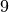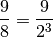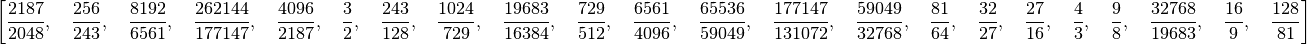# Utilities¶

The PyTuning package contains some utilities which may be useful. In general these tend to be smaller utilities and tasks that are useful in the analysis of musical scales, but they are not full-featured “things” in and of themselves.

## Interval Normalization¶

As an example, the intervalwould normalize to, because 9 needs to be scaled down by three octaves to fall within the limit of 1 and 2:ni = normalize_interval(sp.Integer(9))
print(ni)
9/8


One can also normalize on a non-standard interval, for example, 3:

ni = normalize_interval(sp.Integer(34), octave=3)
print(ni)
34/27


## Distinct Intervals¶

distinct_intervals() returns all the distinct intervals within a musical scale. Note, though, that it does not include the unison (or the octave) in the results, as all scales contain those intervals by definitions.

As an example, if we were to take a Pythagorean scale and find the intervals that exist within it:

pythag = create_pythagorean_scale()
di = distinct_intervals(pythag)


we end up with:## Converting a Ratio to a Cent Value¶

This function is useful if you have a symbolic value (a rational or transcendental, for example) and you want to see its value in cents (a logarithmic scale in which there are 1200 steps in a factor of two). For example:

interval = sp.Rational(3,2) # A perfect fifth
cents = ratio_to_cents(interval)
print(cents)
701.955000865387


## Converting a Cent Value to a Ratio¶

This function takes a cent value and returns it as a frequency ratio (a sympy floating point number).

print(cents_to_ratio(700.0))
1.49830707687668


(In other words, the 12-EDO fifth (700 cents) is very close to that of the Pythagorean fifth (, or 1.5).)

## Converting a Note Number to a Frequency¶

With this function we can calculate the frequency of any note number. If defaults to the MIDI standard, which pegs note number 69 to 440 Hz and uses a 12-EDO scale.

As an example, MIDI note 60 (Middle-C):

print(note_number_to_freq(60))
261.625565300599


But if, for example, we wanted to use a different pitch standard, we could peg A to 444 Hz.

print(note_number_to_freq(60, reference_frequency=444.0))
264.003979530604


You can also pass in a non-EDO tuning if you’re converting a different kind of scale to frequencies. This is used often in the code associated with the tuning tables.

## Naming A Ratio¶

This function will look up the name of a ratio and return it (returning None) if it is not found.

As an example:

pythag = create_pythagorean_scale()
names = [ratio_to_name(x) for x in pythag]


names now contains:

 ['Unison',
'Pythagorean Minor Second',
'Pythagorean Major Second',
'Pythagorean Minor Third',
'Pythagorean Major Third',
'Perfect Fourth',
'Pythagorean Diminished Fifth',
'Perfect Fifth',
'Pythagorean Minor Sixth',
'Pythagorean Major Sixth',
'Pythagorean Minor Seventh',
'Pythagorean Major Seventh',
'Octave']


There are currently about 260 intervals in the internal catalog, so while not complete, the database is fairly extensive.

## Comparing Two Scales¶

This function will produce a simple textual representation of the difference between two scales. As an example, comparing the 12-EDO and Pythagorean scales:

from pytuning.scales import create_edo_scale, create_pythagorean_scale
from pytuning.utilities import compare_two_scales

scale_1 = create_edo_scale(12)
scale_2 = create_pythagorean_scale()

compare_two_scales(scale_1, scale_2, title=['12-TET', 'Pythagorean'])


produces:

          12-TET              Pythagorean
Cents       Freq      Cents       Freq  Delta(Cents)
=========  =========  =========  =========  ============
0.0000   220.0000     0.0000   220.0000        0.0000
100.0000   233.0819    90.2250   231.7695        9.7750
200.0000   246.9417   203.9100   247.5000       -3.9100
300.0000   261.6256   294.1350   260.7407        5.8650
400.0000   277.1826   407.8200   278.4375       -7.8200
500.0000   293.6648   498.0450   293.3333        1.9550
600.0000   311.1270   588.2700   309.0261       11.7300
700.0000   329.6276   701.9550   330.0000       -1.9550
800.0000   349.2282   792.1800   347.6543        7.8200
900.0000   369.9944   905.8650   371.2500       -5.8650
1000.0000   391.9954   996.0900   391.1111        3.9100
1100.0000   415.3047  1109.7750   417.6562       -9.7750
1200.0000   440.0000  1200.0000   440.0000        0.0000